## Projection methods in linear algebra numerics

Linear algebra classes often jump straight to the definition of a projector (as a matrix) when talking about orthogonal projections in linear spaces. As often as it happens, it is not clear how that definition arises. This is what is covered in this post.

## Orthogonal projection: how to build a projector

### Case 1 – 2D projection over (1,0)

It is quite straightforward to understand that orthogonal projection over (1,0) can be practically achieved by zeroing out the second component of any 2D vector, at last if the vector is expressed with respect to the canonical basis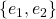. Albeit an idiotic statement, it is worth restating: the orthogonal projection of a 2D vector amounts to its first component alone.

How can this be put math-wise? Since we know that the dot product evaluates the similarity between two vectors, we can use that to extract the first component of a vector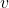. Once we have the magnitude of the first component, we only need to multiply that by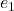itself, to know how much in the direction ofwe need to go. For example, starting from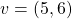, first we get the first component as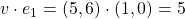; then we multiply this value by e_1 itself: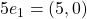. This is in fact the orthogonal projection of the original vector. Writing down the operations we did in sequence, with proper transposing, we get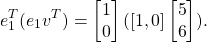One simple and yet useful fact is that when we project a vector, its norm must not increase. This should be intuitive: the projection process either takes information away from a vector (as in the case above), or rephrases what is already there. In any way, it certainly does not add any. We may rephrase our opening fact with the following proposition:

PROP 1: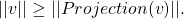This is can easily be seen through the pitagorean theorem (and in fact only holds for orthogonal projection, not oblique):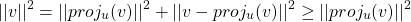## Diagonalizing a matrix NOT having full rank: what does it mean?

This is going to be a quick intuition about what it means to diagonalize a matrix that does not have full rank (i.e. has null determinant).

Every matrix can be seen as a linear map between vector spaces. Stating that a matrix is similar to a diagonal matrix equals to stating that there exists a basis of the source vector space in which the linear transformation can be seen as a simple stretching of the space, as re-scaling the space. In other words, diagonalizing a matrix is the same as finding an orthogonal grid that is transformed in another orthogonal grid. I recommend this article from AMS for good visual representations of the topic.

### Diagonalization on non full rank matrices

That’s all right – when we have a matrix from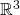in, if it can be diagonalized, we can find a basis in which the transformation is a re-scaling of the space, fine.

But what does it mean to diagonalize a matrix that has null determinant? The associated transformations have the effect of killing at least one dimension: indeed, axmatrix of rank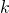has the effect of lowering the output dimension by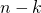. For example, a 3×3 matrix of rank 2 will have an image of size 2, instead of 3. This happens because two basis vectors are merged in the same vector in the output, so one dimension is bound to collapse.

Let’s consider the sample matrix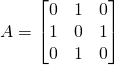which has non full rank because has two equal rows. Indeed, one can check that the two vectorsgo in the same basis vector. This means thatinstead of 3. In fact, it is common intuition that when the rank is not full, some dimensions are lost in the transformation. Even if it’s a 3×3 matrix, the output only has 2 dimensions. It’s like at the end of Interstellar when the 4D space in which Cooper is floating gets shut.

However,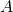is also a symmetric matrix, so from the spectral theorem we know that it can be diagonalized. And now to the vital questions: what do we expect? What meaning does it have? Do we expect a basis of three vectors even if the map destroys one dimension?

Pause and ponder.

## Overdetermined and underdetermined systems of equations put simply

This article aims at providing real world examples and links for overdetermined and undetermined systems of equations. Before starting, we will suppose that all over and underdetermined systems are obtained from square systems which admit one and only one solution (i.e. comes from a coefficient matrix with non zero determinant).

## Overdetermined systems

When a system of linear equations has more equations than unknowns, we say it is overdetermined. It means what it says: too many rules at once are being imposed, and some of them may be conflicting. Still, it is false to say that an overdetermined system does not have any solutions: it may or it may not.

### Intuition

Intuitively, we can think of a system of equations as a set of requests. Imagine you have a group ofpeople in front of you (the unknowns), and you are supposed to give each person something specific to do. If you give more commands than the number of people, then we have an overdetermined system. It is clear that when this happens, at least one person must have received more than one command. However, there are two possible scenarios.

## Quick method to find line of shortest distance for skew lines

In linear algebra it is sometimes needed to find the equation of the line of shortest distance for two skew lines. What follows is a very quick method of finding that line.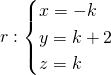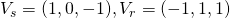So they clearly aren’t parallel. They aren’t incidental as well, because the only possible intersection point is for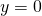, but when,is at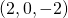, which doesn’t belong to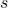. It does indeed make sense to look for the line of shortest distance between the two, confident that we will find a non-zero result.